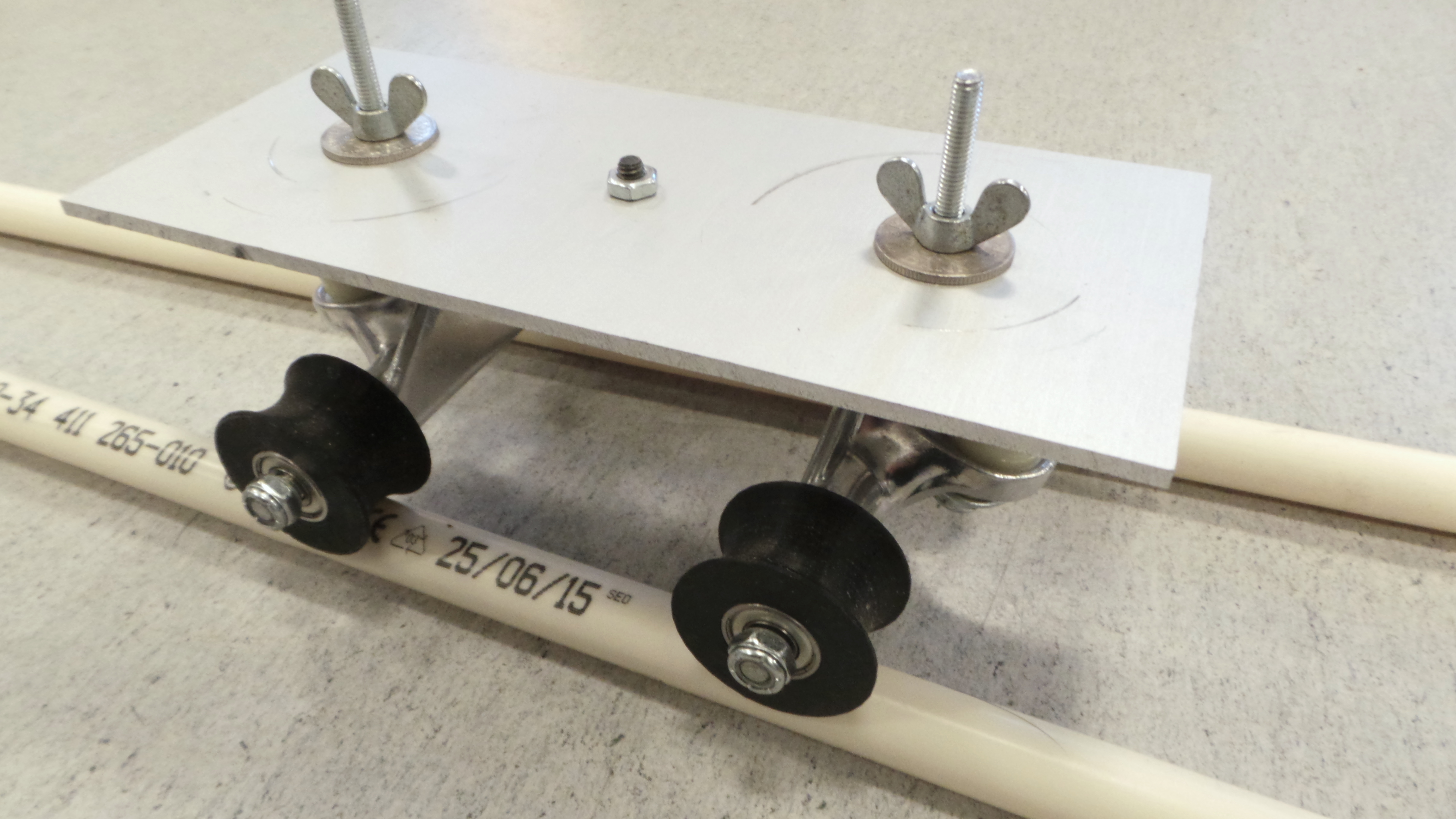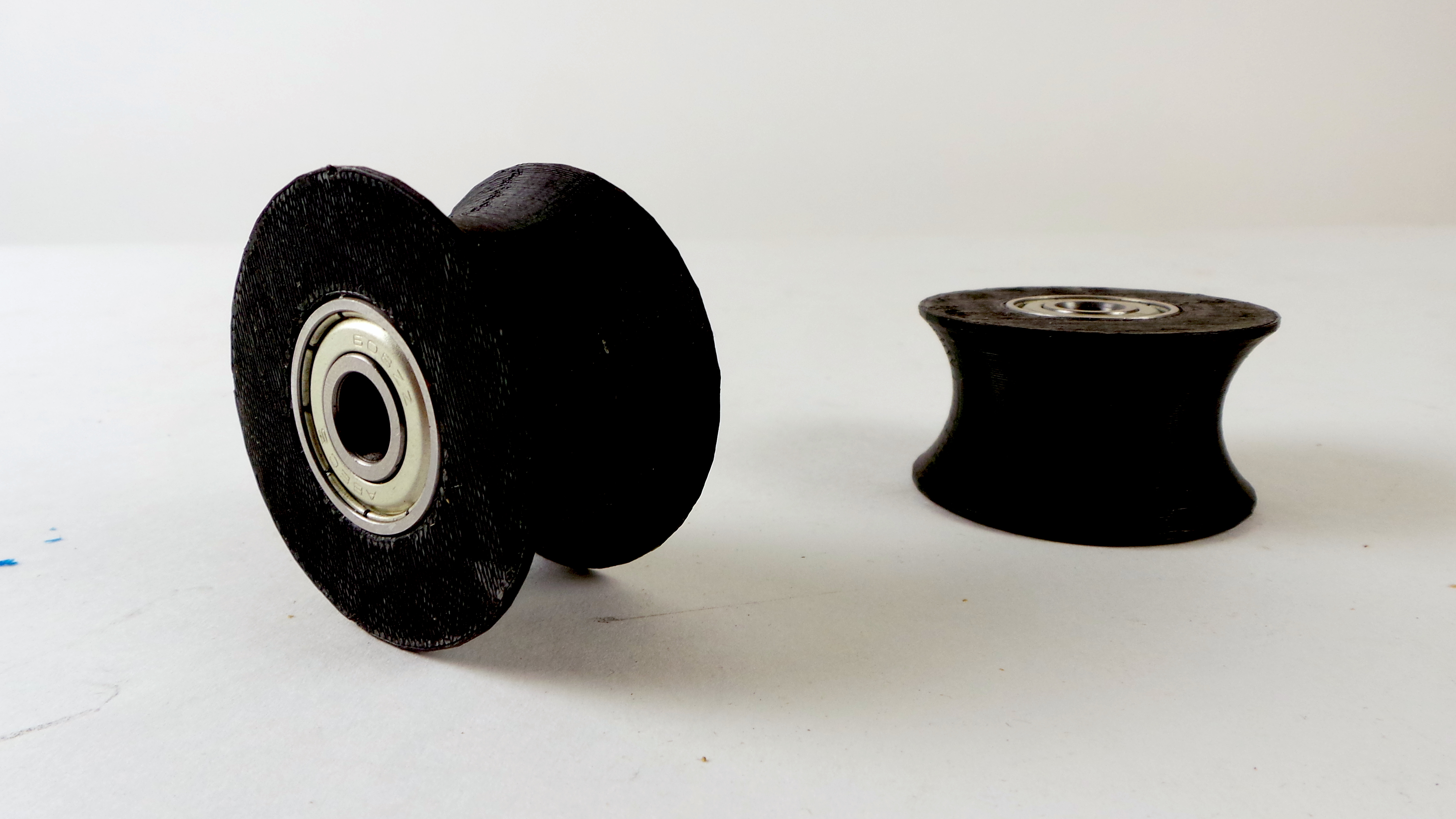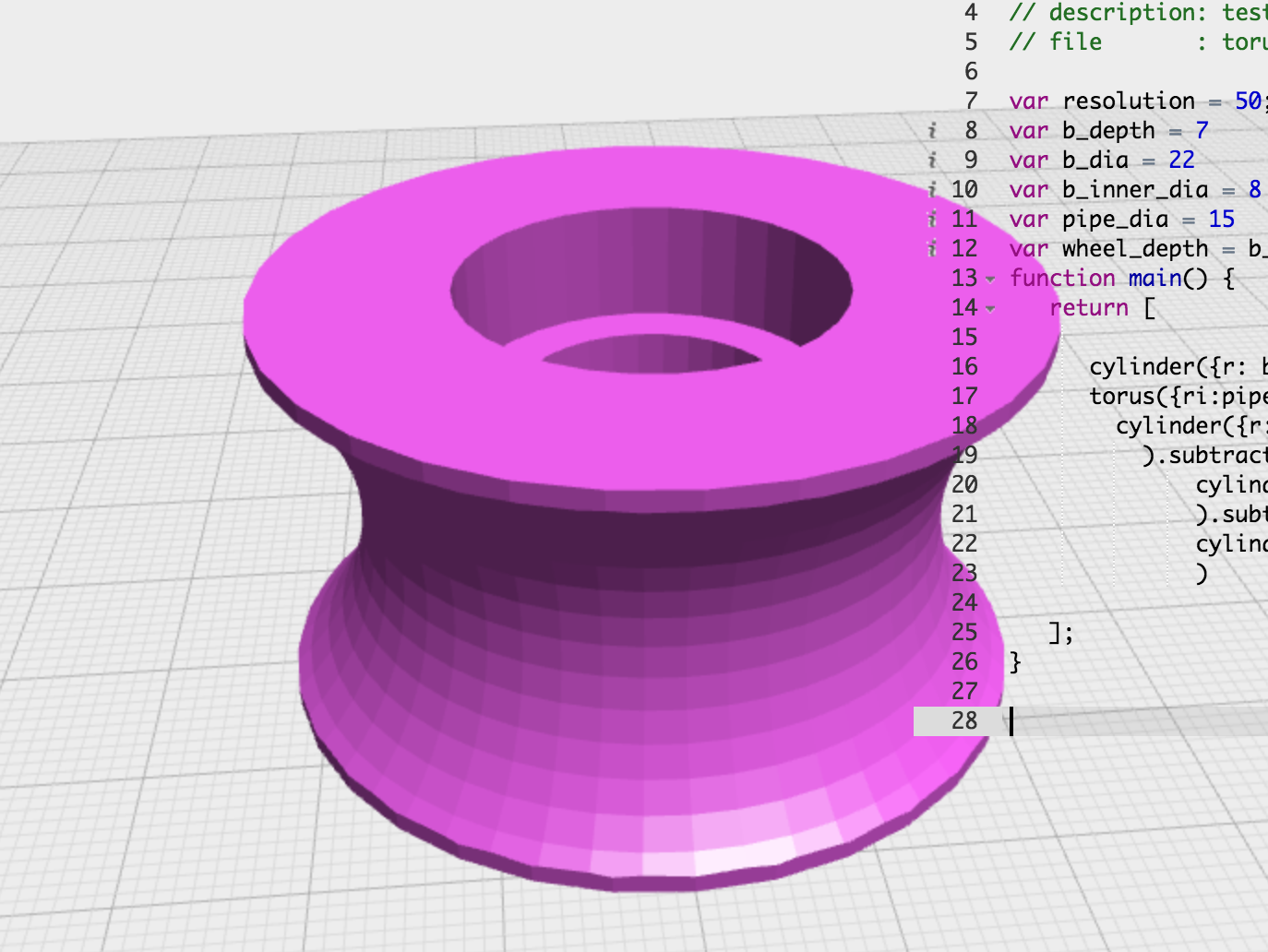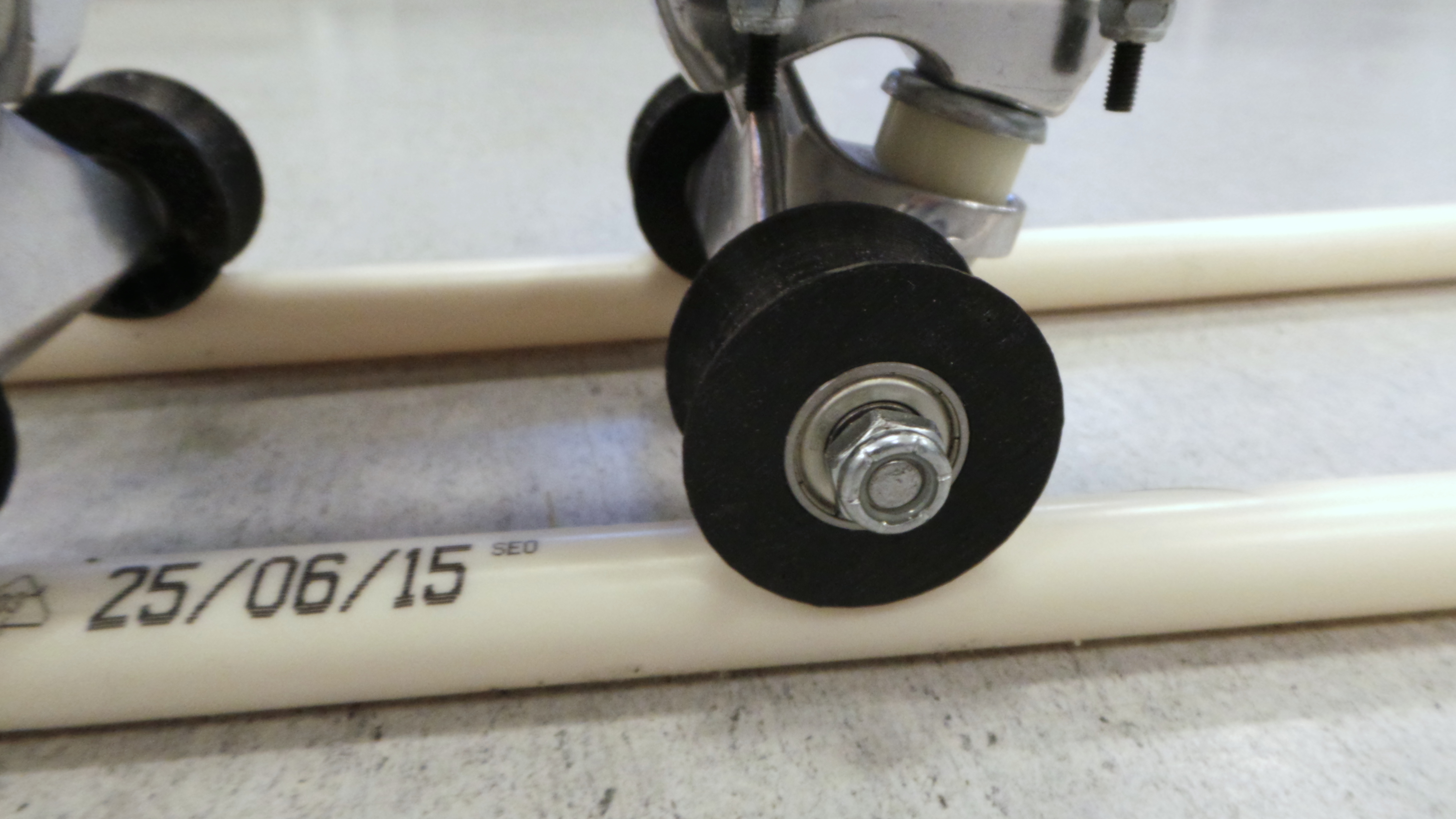Published: January 05, 2016, Edited by: Mads Hobye

# 3D print U wheels for a dollyTo make a simple dolly slider design e.g. for timelapse or for smooth movements we have designed u wheels for you to 3D print.

The dolly itself consists of two skateboard trucks mounted on an aluminium plate.Use OpenJSCad and copy-paste the code below into it. Then press shift+enter to compile the code into a 3D shape. You can change parameters to take different pipe diameters into account.``````var resolution = 50;
var b_depth = 7
var b_dia = 22
var b_inner_dia = 8
var pipe_dia = 15
var wheel_depth = b_depth*3.5
function main() {
return [

cylinder({r: b_dia, h:wheel_depth}).subtract(
torus({ri:pipe_dia/2,ro:b_dia+10, fni:resolution,fno:resolution}).translate([0,0,wheel_depth/2])).subtract(
cylinder({r: b_inner_dia/2+4, h: wheel_depth})
).subtract(
cylinder({r: b_dia/2, h: b_depth}).translate([0,0,wheel_depth-b_depth])
).subtract(
cylinder({r: b_dia/2, h: b_depth}).translate([0,0,0])
)

];
}
``````